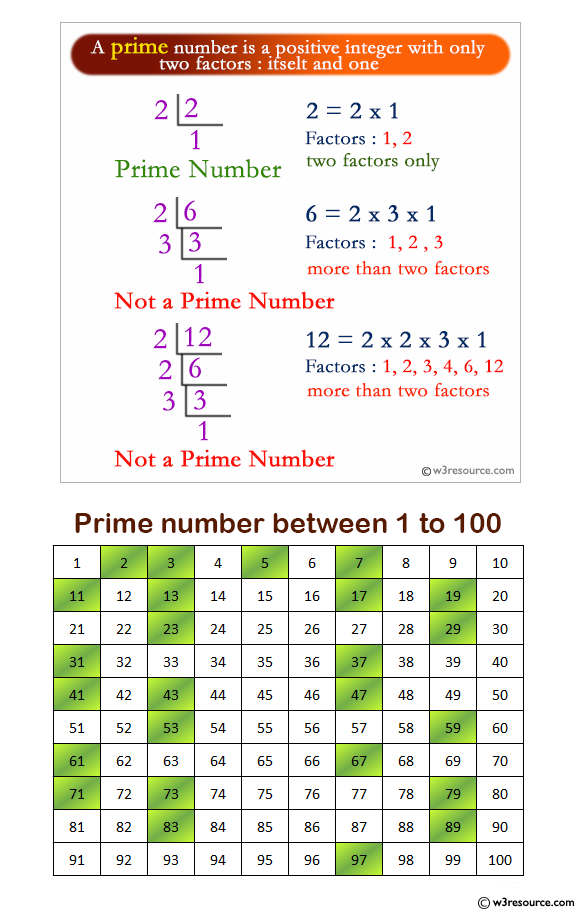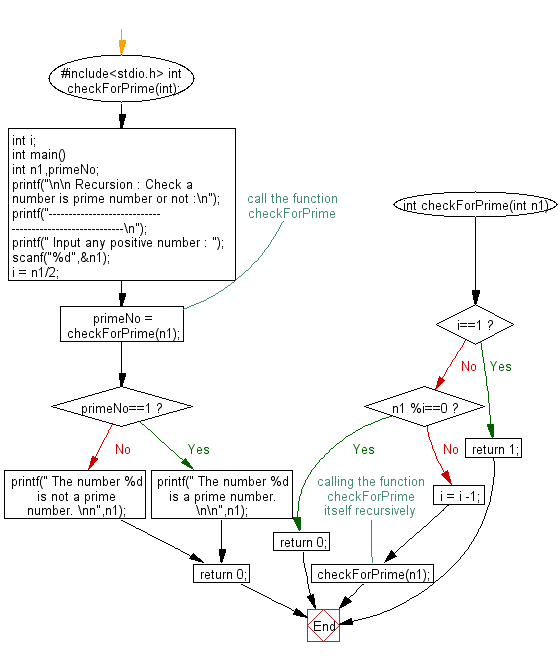﻿ C Program: Check a number is a prime number or not - w3resource

# C Exercises: Check a number is a prime number or not

## C Recursion : Exercise-12 with Solution

Write a program in C to check if a number is a prime number or not using recursion.

Pictorial Presentation:Sample Solution:

C Code:

``````#include

int checkForPrime(int);
int i;

int main()
{

int n1,primeNo;

printf("\n\n Recursion : Check a number is prime number or not :\n");
printf("--------------------------------------------------------\n");

printf(" Input any positive number : ");
scanf("%d",&n1);

i = n1/2;

primeNo = checkForPrime(n1);//call the function checkForPrime

if(primeNo==1)
printf(" The number %d is a prime number. \n\n",n1);
else
printf(" The number %d is not a prime number. \nn",n1);
return 0;
}

int checkForPrime(int n1)
{
if(i==1)
{
return 1;
}
else if(n1 %i==0)
{
return 0;
}
else
{
i = i -1;
checkForPrime(n1);//calling the function checkForPrime itself recursively
}
}
```
```

Sample Output:

``` Recursion : Check a number is prime number or not :
--------------------------------------------------------
Input any positive number : 7
The number 7 is a prime number.
```

Explanation:

```int checkForPrime(int n1)
{
if(i==1)
{
return 1;
}
else if(n1 %i==0)
{
return 0;
}
else
{
i = i -1;
checkForPrime(n1);//calling the function checkForPrime itself recursively
}
}
```

The function ‘checkForPrime()’ takes an integer n1 as input and checks if the number is divisible by i (which is initially set to some value outside the function).

• If i is equal to 1, the function returns 1, indicating that the number is prime.
• If n1 is divisible by i, the function returns 0, indicating that the number is not prime.
• Otherwise, the function decrements the value of i and calls itself recursively with the same value of n1.
• The function continues to call itself recursively until i reaches 1 or n1 is found to be divisible by i.
• Time complexity and space complexity:

The time complexity of this function is O(n) where n is the input number since the function checks all the numbers from 2 to n-1 to determine if the number is prime or not.

The space complexity is O(1) since the function uses only constant space for storing the value of i.

Flowchart:C Programming Code Editor:

Have another way to solve this solution? Contribute your code (and comments) through Disqus.

﻿

## C Programming: Tips of the Day

__FILE__ macro shows full path

```#include <string.h>

#define __FILENAME__ (strrchr(__FILE__, '/') ? strrchr(__FILE__, '/') + 1 : __FILE__)
For Windows use '\\' instead of '/'.
```

Ref : https://bit.ly/3iEWRoT

We are closing our Disqus commenting system for some maintenanace issues. You may write to us at reach[at]yahoo[dot]com or visit us at Facebook

Test your Programming skills with w3resource's quiz.

﻿

## C Programming: Tips of the Day

__FILE__ macro shows full path

```#include <string.h>

#define __FILENAME__ (strrchr(__FILE__, '/') ? strrchr(__FILE__, '/') + 1 : __FILE__)
For Windows use '\\' instead of '/'.
```

Ref : https://bit.ly/3iEWRoT

We are closing our Disqus commenting system for some maintenanace issues. You may write to us at reach[at]yahoo[dot]com or visit us at Facebook